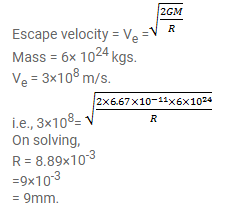# A mass of 6 × 1024 kg (equal to the mass of the earth)

Question:

A mass of $6 \times 1024 \mathrm{~kg}$ (equal to the mass of the earth) is to be compressed in a sphere in such a way that the escape velocity from its surface is $3 \times 108 \mathrm{~m} / \mathrm{s}$. What should be the radius of the sphere?

Solution: